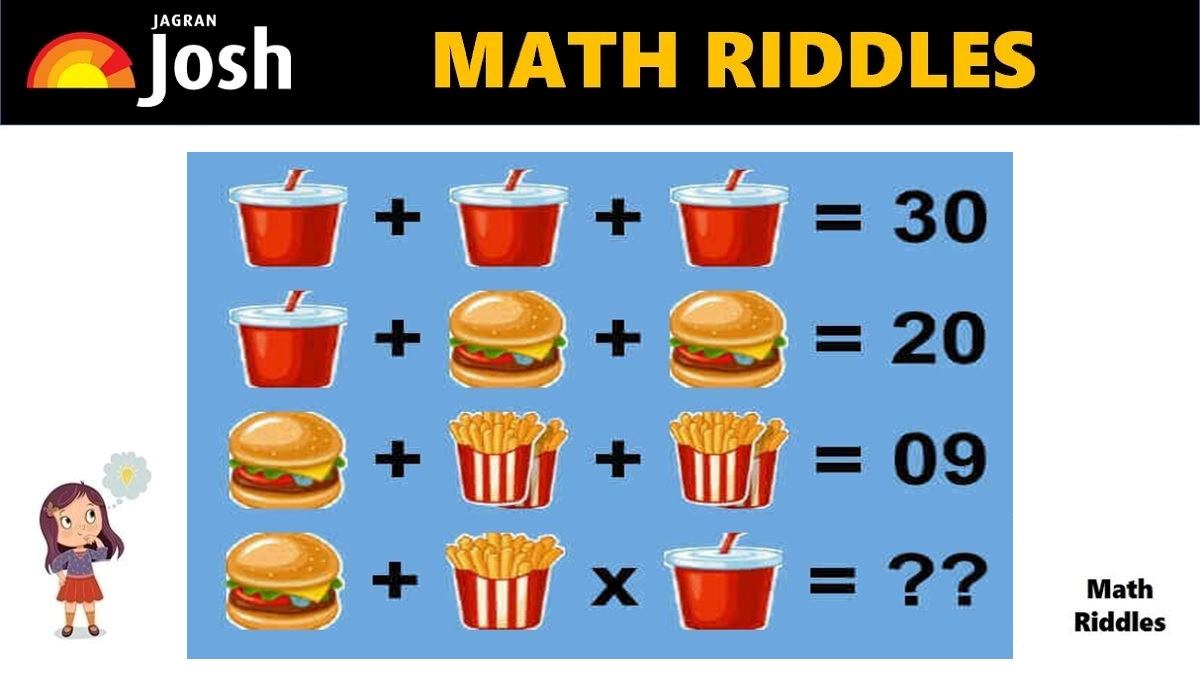# Math Riddles With Answers: Can You Find The Value of Burger, Fries, Coke in 20 Seconds?

Are you a math genius? Only 1% with a high IQ can solve this tricky burger, fries, coke’ math picture puzzle in 20 seconds.Math Riddles With Answers: Can You Find The Value of Burger, Fries, Coke in 20 Seconds?

Try to solve this ‘Coke, Burger, Fries’ Math Puzzle. This math puzzle is for genius only. This math riddles which definitely looks tempting as a McDonalds’s burgers, fries, and coke combo has stumped the internet. Many people tried to find the value of each burger, fries, and coke to solve this math riddle. Are you a genius to find the value within 20 seconds? Enjoy solving this math riddles, if you find the correct answer then treat yourself with a combo of burger, fries, and coke.

## Math Riddles with Answers: Solution

Row 1: There are 3 glasses of coke which equal to 30.

So, we get 3 cokes = 30, if we divide both sides (LHS and RHS) by 3 then we get the result that value of 1 coke is equal to 10. Now, we will use this value of 1 coke to solve the next row of the problem. See picture below to understand better.

Math Riddles With Answers: Can You Find The Value of Burger and Beer in 20 Seconds?

Picture Puzzles Can You Find The Hidden Dice Among The Cheese and Mice In 11 Seconds?

Row 2: Now, we have 1 coke + 1 burger + 1 burger = 20. We know that 1 coke equals 10, so we apply the value and solve the equation to find the value of 1 burger. See picture below to understand better.

So, now we know the value of 1 burger is 5. Let us find out the value of Fries.

Picture Puzzle: How Sharp Is Your Vision? Find Hidden Animals In The Picture In 11 Seconds

Math Riddles With Answers: Can You Solve This Tricky Math Rebus Puzzle 20 Seconds?

Row 3: Now, we got 1 burger + 1 Pair of Fries + 1 Pair of Fries = 9

We already know that value of 1 burger is 5. So, we apply the value of 1 burger to the equation and figure out the value of 1 Fries. See picture below to understand better.

Row 4: Now, we got 1 burger + 1 Single Packet of Fries + 1 Coke = ??

Now, we have figured out the values of each item in our previous equations, we shall apply the values to find the final answer.

=> 1 burger + 1 Single Packet of Fries + 1 Coke = ??

=> 5 + 1 x 10 = ??

Applying BODMAS (Bracket, Of, Division, Multiplication, Addition, and Subtraction)

=> 5 + 10 = 15

Great Work!

## Tell us in comments: Did you solve this math puzzle correctly in 20 seconds?

Check out more amazing tricky math puzzles!

Math Riddles With Answers: Can You Find The Value of Each Fruit in 20 Seconds?

Picture Puzzle: Find Two Same Emojis In 11 Seconds, 99% People Failed To Find Them

Picture Puzzle: Only 1% Genius Can Find The Hidden Words In The Picture in 11 seconds

Picture Puzzle: Find the Mistake In 11 Seconds, High Observational Skills Required

Picture Puzzle: Test Your Intelligence Level, 1% Genius Can Spot All 6 Hidden Words in 11 Seconds

Get the latest General Knowledge and Current Affairs from all over India and world for all competitive exams.
खेलें हर किस्म के रोमांच से भरपूर गेम्स सिर्फ़ जागरण प्ले पर